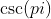Trigonometry : Determine Which Values of Trigonometric Functions are Undefined

Example Questions

← Previous 1

Example Question #1 : Determine Which Values Of Trigonometric Functions Are Undefined

For which values of, where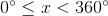in the unit circle, is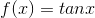undefined?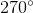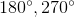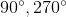Explanation:

Recall that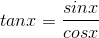. Since the ratio of any two real numbers is undefined when the denominator is equal tomust be undefined for those values ofwhere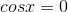. Restricting our attention to those values ofbetween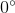and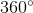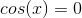when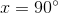or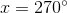. Hence,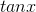is undefined whenor.

Example Question #2 : Determine Which Values Of Trigonometric Functions Are Undefined

What is the domain of f(x) = sin x?

All negative numbers and 0

All positive numbers and 0

All real numbers except 0

All real numbers

All real numbers

Explanation:

The domain of a function is the range of all possible inputs, or x-values, that yield a real value for f(x). Trigonometric functions are equal to 0, 1, -1 or undefined when the angle lies on an axis, meaning that the angle is equal to 0, 90, 180 or 270 degrees (0, (pi)/2, pi or 3(pi)/2 in radians.) Trigonometric functions are undefined when they represent fractions with denominators equal to zero. Sine is defined as the ratio between the side length opposite to the angle in question and the hypotenuse (SOH, or sin x = opposite/hypotenuse). In any triangle created by the angle x and the x-axis, the hypotenuse is a nonzero number. As a result, the denominator of the fraction created by the definition sin x = opposite/hypotenuse is not equal to zero for any angle value x. Therefore, the domain of f(x) = sin x is all real numbers.

Example Question #3 : Determine Which Values Of Trigonometric Functions Are Undefined

What is the domain of f(x) = cos x?

All positive numbers and 0

All real numbers

All negative numbers and 0

All real numbers except 0

All real numbers

Explanation:

The domain of a function is the range of all possible inputs, or x-values, that yield a real value for f(x). Trigonometric functions are equal to 0, 1, -1 or undefined when the angle lies on an axis, meaning that the angle is equal to 0, 90, 180 or 270 degrees (0, (pi)/2, pi or 3(pi)/2 in radians.) Trigonometric functions are undefined when they represent fractions with denominators equal to zero. Cosine is defined as the ratio between the side length opposite to the angle in question and the hypotenuse (CAH, or cos x = adjacent/hypotenuse). In any triangle created by the angle x and the x-axis, the hypotenuse is a nonzero number. As a result, the denominator of the fraction created by the definition cos x = adjacent/hypotenuse is not equal to zero for any angle value x. Therefore, the domain of f(x) = cos x is all real numbers.

Example Question #4 : Determine Which Values Of Trigonometric Functions Are Undefined

Which of the following trigonometric functions is undefined?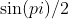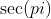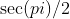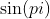Explanation:

Trigonometric functions are equal to 0, 1, -1 or undefined when the angle lies on an axis, meaning that the angle is equal to 0, 90, 180 or 270 degrees (0, (pi)/2, pi or 3(pi)/2 in radians.) Trigonometric functions are undefined when they represent fractions with denominators equal to zero. Secant is the reciprocal of cosine, so the secant of any angle x for which cos x = 0 must be undefined, since it would have a denominator equal to 0. The value of cos (pi/2) is 0, so the secant of (pi)/2 must be undefined.

Example Question #5 : Determine Which Values Of Trigonometric Functions Are Undefined

Which of the following trigonometric functions is undefined?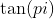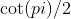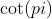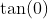Explanation:

Trigonometric functions are equal to 0, 1, -1 or undefined when the angle lies on an axis, meaning that the angle is equal to 0, 90, 180 or 270 degrees (0, (pi)/2, pi or 3(pi)/2 in radians.) Trigonometric functions are undefined when they represent fractions with denominators equal to zero. Cotangent is the reciprocal of tangent, so the cotangent of any angle x for which tan x = 0 must be undefined, since it would have a denominator equal to 0. The value of tan (pi) is 0, so the cotangent of (pi) must be undefined.

Example Question #6 : Determine Which Values Of Trigonometric Functions Are Undefined

Which of the following trigonometric functions is undefined?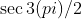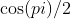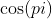Explanation:

Trigonometric functions are equal to 0, 1, -1 or undefined when the angle lies on an axis, meaning that the angle is equal to 0, 90, 180 or 270 degrees (0, (pi)/2, pi or 3(pi)/2 in radians.) Trigonometric functions are undefined when they represent fractions with denominators equal to zero. Secant is the reciprocal of cosine, so the secant of any angle x for which cos x = 0 must be undefined, since it would have a denominator equal to 0. The value of cos 3(pi/2) is 0, so the secant of 3(pi)/2 must be undefined.

Example Question #7 : Determine Which Values Of Trigonometric Functions Are Undefined

Which of the following trigonometric functions is undefined?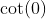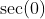Explanation:

Trigonometric functions are equal to 0, 1, -1 or undefined when the angle lies on an axis, meaning that the angle is equal to 0, 90, 180 or 270 degrees (0, (pi)/2, pi or 3(pi)/2 in radians.) Trigonometric functions are undefined when they represent fractions with denominators equal to zero. Cotangent is the reciprocal of tangent, so the cotangent of any angle x for which tan x = 0 must be undefined, since it would have a denominator equal to 0. The value of tan (0) is 0, so the cotangent of (0) must be undefined.

Example Question #8 : Determine Which Values Of Trigonometric Functions Are Undefined

Which of the following trigonometric functions is undefined?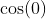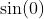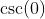Explanation:

Trigonometric functions are equal to 0, 1, -1 or undefined when the angle lies on an axis, meaning that the angle is equal to 0, 90, 180 or 270 degrees (0, (pi)/2, pi or 3(pi)/2 in radians.) Trigonometric functions are undefined when they represent fractions with denominators equal to zero. Cosecant is the reciprocal of sine, so the cosecant of any angle x for which sin x = 0 must be undefined, since it would have a denominator equal to 0. The value of sin (0) is 0, so the cosecant of 0 must be undefined.

Example Question #9 : Determine Which Values Of Trigonometric Functions Are Undefined

Which of the following trigonometric functions is undefined?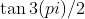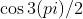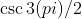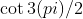Explanation:

Trigonometric functions are equal to 0, 1, -1 or undefined when the angle lies on an axis, meaning that the angle is equal to 0, 90, 180 or 270 degrees (0, (pi)/2, pi or 3(pi)/2 in radians.) Trigonometric functions are undefined when they represent fractions with denominators equal to zero. Tangent is defined as the ratio between the side length opposite to the angle in question and the side length adjacent to it (TOA, or tan x = opposite/adjacent). In a triangle created by the angle x and the x-axis, the adjacent side length lies along the x-axis; however, when the angle x lies on the y-axis, no length can be drawn along the x-axis to represent the angle. As a result, the denominator of the fraction created by the definition tan x = opposite/adjacent is equal to zero for any angle along the y-axis (90 or 270 degrees, or pi/2 or 3pi/2 in radians.) Therefore, tan 3(pi)/2 is undefined.

Example Question #10 : Determine Which Values Of Trigonometric Functions Are Undefined

Which of the following trigonometric functions is undefined?